# 31 Year NEET Previous Year Questions: Nuclei - 2

## 30 Questions MCQ Test Topic-wise MCQ Tests for NEET | 31 Year NEET Previous Year Questions: Nuclei - 2

Description
Attempt 31 Year NEET Previous Year Questions: Nuclei - 2 | 30 questions in 60 minutes | Mock test for NEET preparation | Free important questions MCQ to study Topic-wise MCQ Tests for NEET for NEET Exam | Download free PDF with solutions
QUESTION: 1

### The activity of a radioactive sample is measuredas 9750 counts per minute at t = 0 and as 975counts per minute at t = 5 minutes. The decayconstant is approximately 

Solution: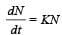9750 = KN0 ............. (1)
975 = KN ............. (2)
Dividing (1) by (2)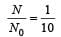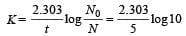= 0.4606 = 0.461 per minute

QUESTION: 2

### The stable nucleus that has a radius half that of Fe56 is 

Solution:

The nuclear radius R = RoA1/3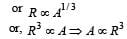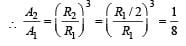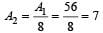QUESTION: 3

### In a fission reaction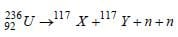the binding energy per nucleon of X and Y is 8.5MeV whereas of 236U is 7.6 MeV. The totalenergy liberated will be about 

Solution:

Binding energy
= 117 × 8.5 + 117 × 8.5 – 236 × 7.6
= 234 × 8.5 – 236 × 7.6
= 1989 – 1793.6 = 200 MeV
Thus, in per fission of Uranium nearly 200
MeV energy is liberated

QUESTION: 4

A free neutron decays into a proton, an electronand 

Solution: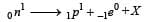X must have zero charge and almost zero mass as electron  is emitted. Hence X must be antineutrino.

QUESTION: 5

The most penetrating radiation of the following is       

Solution:

The penetrating power of radiation is directly proportional to the energy of its photon.
Energy of a photon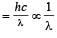∴ Penetrating power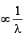λ is minimum for γ-rays, so penetrating power is maximum of γ-rays.

QUESTION: 6

Which of the following is used as a moderator innuclear reactors? 

Solution:

Moderator used in nuclear r eactor are graphite and heavy water.

QUESTION: 7

Half-lives of two radioactive substances A and B are respectively 20 minutes and 40 minutes.Initially, the samples of A and B have equalnumber of nuclei. After 80 minutes the ratio ofremaining numbers of A and B nuclei is 

Solution:

80 = 20 × nA ⇒ nA = 4
80 = 40 × nB ⇒ nB = 2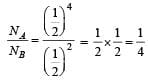QUESTION: 8

Atomic weight of Boron is 10.81 and it has two isotopes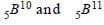. Then the ratio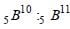in nature would be        

Solution:

Suppose that, The number of 10B type atoms = x and the number of 11B type atoms = y
Weight of 10B type atoms = 10x
Weight of 11B type atoms = 11y
Total number of atoms = x + y

∴  Atomic weight⇒10x + 11y = 10.81x + 10.81y
⇒ 0.81x = 0.19y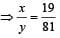QUESTION: 9

A nucleus nXm emits one α and two β particles. The resulting nucleus is

Solution: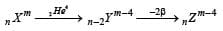QUESTION: 10

Complete the equation for the following fission process :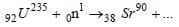Solution: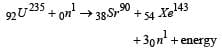QUESTION: 11

Alpha-particles are 

Solution:

We know that alpha particles are the nucleus of ionized helium atoms which contain two protons and two neutrons. These are emitted by the nuclei of certain radioactive substances. Streams of alpha particles, called α-rays, produce intense ionisation in gases through which they pass and are easily absorbed by matter.

QUESTION: 12

After 1α and 2 β-emissions 

Solution:

Emission of 1α particle led to decrease in atomic number by 2 while mass number by 4.
On the other hand, emission of 2β particles increases  atomic number by 2. Hence, overall emission of, 1α and 2β particles led to decrease in mass number by 4.

QUESTION: 13

The decay constant (λ) and the half-life (T) of a radioactive isotope are related as 

Solution: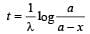when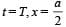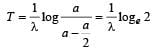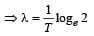QUESTION: 14

Atomic hydrogen has life period of 

Solution:

Atomic hydrogen is unstable and it has life period of a fraction of a second.

QUESTION: 15

It is possible to understand nuclear fission onthe basis of the 

Solution:

According to liquid drop model of nucleus, an excited nucleus breaks into lighter nuclei just like an excited drop breaks into tiny drops.

QUESTION: 16

In the following nuclear reaction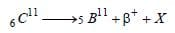what does X stand for? 

Solution: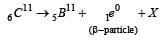X is a neutrino, when β-particle is emitted.

QUESTION: 17

Mn and Mp represent mass of neutron and protonrespectively. If an element having atomic massM has N-neutron and Z-proton, then the correctrelation will be 

Solution:

Given : Mass of neutron = Mn Mass of proton = Mp; Atomic mass of the element = M ;
Number of neutrons in the element = N and number of protons in the element = Z.
We know that the atomic mass (M) of any stable nucleus is always less than the sum of the masses of the constituent particles.
Therefore, M < [NMn + ZMp].

QUESTION: 18

A sample has 4 × 1016 radioactive nuclei of halflife 10 days. The number of atoms decaying in 30 days is 

Solution: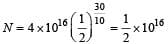Atoms decayed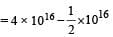= 3.5 × 1016

QUESTION: 19

A deuteron strikes 8O16 nucleus with subsequent emission of an alpha particle. Identify the nucleus so produced 

Solution: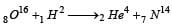QUESTION: 20

For a nuclear fusion process, the suitable nucleiare 

Solution:

For nuclear fusion process the nuclei with low mass are suitable.

QUESTION: 21

Solar energy is mainly caused due to 

Solution:

As a result of fusion, enormous amount of heat is liberated which is the main cause of source of solar energy.

QUESTION: 22

A nuclear reaction is given by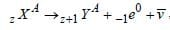represents

Solution:

-1e0 represents a β-decay..

QUESTION: 23

A sample of radioactive element has a mass of 10gm at an instant t=0. The approximate mass ofthis element in the sample after two mean lives is 

Solution:

Using the relation for mean life.
Given :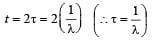Then from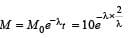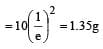QUESTION: 24

The mass number of a nucleus is 

Solution:

In case of hydrogen atom, Mass number = atomic number

QUESTION: 25

The mass of proton is 1.0073 u and that ofneutron is 1.0087 u (u = atomic mass unit). Thebinding energy of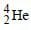is    

Solution:

Δm = (2 × 1.0074 + 2 × 1.0087 – 4.0015) = 0.0307
E = (Δm) × 931 MeV = 0.0307 × 931 = 28.5 MeV

QUESTION: 26

The volume occupied by an atom is greater thanthe volume of the nucleus by a factor of about

Solution: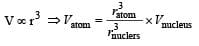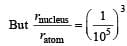Hence, Vatom = 1015 × Vnucleus

QUESTION: 27

If in nuclear fusion process the masses of thefusing nuclei be m1 and m2 and the mass of theresultant nucleus be m3, then 

Solution:

m3 < (m1 + m2) (∵ m1 + m2 = m3 + E ]
as E = [m1 + m2 – m3] C2

QUESTION: 28

A nucleus represented by the symbol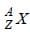has

Solution: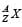has Z protons and (A – Z) neutrons

QUESTION: 29

Mp denotes the mass of a proton and Mn that ofa neutron. A given nucleus, of binding energyB, contains Z protons and N neutrons. The mass M (N, Z) of the nucleus is given by (c is thevelocity of light) 

Solution:

Mass defect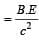Mass of nucleus = Mass of proton + mass of neutron  – mass defect

QUESTION: 30

The nuclei of which one of the following pairs of nuclei are isotones? 

Solution:

Isotones means equal number of neutrons i.e.,   (A–Z) = 74 –34 = 71 – 31 = 40.Use Code STAYHOME200 and get INR 200 additional OFF Use Coupon Code